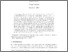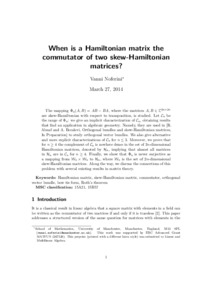# When is a Hamiltonian matrix the commutator of two skew-Hamiltonian matrices?

Noferini, V (2015) 'When is a Hamiltonian matrix the commutator of two skew-Hamiltonian matrices?' Linear and Multilinear Algebra, 63 (8). 1531 - 1552. ISSN 0308-1087Preview
Text
MIMS_ep2014_15.pdf

Download (546kB) | Preview

## Abstract

The mapping (Formula presented.) , where the matrices (Formula presented.) are skew-Hamiltonian with respect to transposition, is studied. Let (Formula presented.) be the range of (Formula presented.) : we give an implicit characterization of (Formula presented.) , obtaining results that find an application in algebraic geometry. Namely, they are used in [R. Abuaf and A. Boralevi, Orthogonal bundles and skew-Hamiltonian matrices, Submitted] to study orthogonal vector bundles. We also give alternative and more explicit characterizations of (Formula presented.) for (Formula presented.). Moreover, we prove that for (Formula presented.) , the complement of (Formula presented.) is nowhere dense in the set of (Formula presented.) -dimensional Hamiltonian matrices, denoted by (Formula presented.) , implying that almost all matrices in (Formula presented.) are in (Formula presented.) for (Formula presented.). Finally, we show that (Formula presented.) is never surjective as a mapping from (Formula presented.) to (Formula presented.) , where (Formula presented.) is the set of (Formula presented.) -dimensional skew-Hamiltonian matrices. Along the way, we discuss the connections of this problem with several existing results in matrix theory.

Item Type: Article Q Science > QA Mathematics Faculty of Science and Health > Mathematical Sciences, Department of Jim Jamieson 20 Oct 2015 13:28 29 Mar 2021 12:15 http://repository.essex.ac.uk/id/eprint/15326

### Actions (login required)View Item